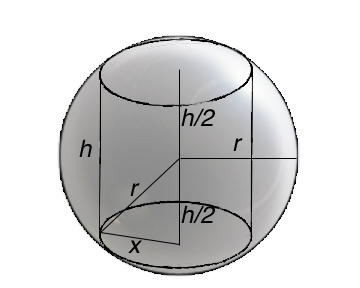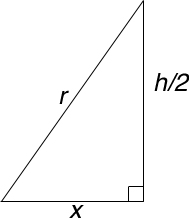SEARCH HOMEMath Central Quandaries & QueriesQuestion from Jim, a student: Hello I have a hard time finishing this question: A right circular cylinder has to be designed to sit inside a sphere of radius 6 meters so that each top and bottom of the cylinder touches the sphere along its complete circular edge. What are the dimensions of the cylinder of max volume and what is the volume?Hi Jim

Using the information you gave me, I came up with the following diagramsThe volume of the cylinder is V=πx2h. The radius of the sphere and the radius of the cylinder's base form a triangle with the height of the cylinder. Using the Pythagorean Theorem we can say x2+(½ h)2=r2

Using implicit differentiation with respect to x, we arrive at the following

 dV/dx = 2πxh + πx2dh/dx 2x+½ h dh/dx = 2r dr/dx

Since the radius is not changing dr/dx = 0

2x+½ h dh/dx = 0

You can now solve for dh/dx from the above equation and substitute it into the change of volume formula

dh/dx = -4x/h

dV/dx = xh + πx2dh/dx= xh + πx2(-4x/h)

At minimum or maximums, rates of change equal zero. So for the maximum volume dV/dx=0

0 = xh + πx2dh/dx= xh + πx2(-4x/h)

You can now solve for x in terms of h then use the Pythagorean Theorem to find the dimensions of your maximum cylinder.

Hope this helps,

JaniceMath Central is supported by the University of Regina and The Pacific Institute for the Mathematical Sciences.